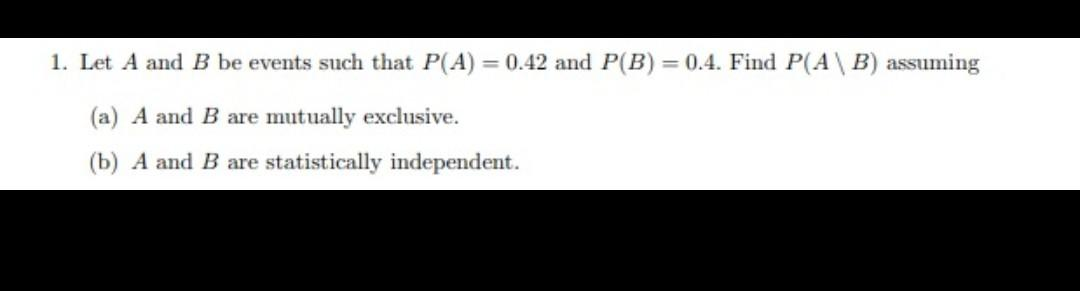# Question Solved1 Answer1. Let A and B be events such that P(A) = 0.42 and P(B) = 0.4. Find P(AB) assuming (a) A and B are mutually exclusive. (b) A and B are statistically independent.9ML8Z3 The Asker · Probability and StatisticsTranscribed Image Text: 1. Let A and B be events such that P(A) = 0.42 and P(B) = 0.4. Find P(AB) assuming (a) A and B are mutually exclusive. (b) A and B are statistically independent.
More
Transcribed Image Text: 1. Let A and B be events such that P(A) = 0.42 and P(B) = 0.4. Find P(AB) assuming (a) A and B are mutually exclusive. (b) A and B are statistically independent.